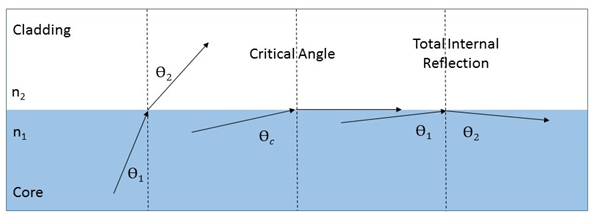# Total Internal Reflection

### FIBER OPTIC BASICS – TOTAL INTERNAL REFLECTION

Long distance transmission of optical signals over fiber optic cables is possible due to the phenomenon of Total Internal Reflection (TIR). When a wave, in this case light, encounters the boundary between materials with different refractive indices, the wave may be partially refracted at the boundary and partially reflected. However, if the angle of incidence is greater than the “critical angle”, the wave will not be refracted but will be totally reflected internally. This will only occur when the wave in a medium with a higher refractive index meets a boundary with a medium of lower refractive index. This is the case in fiber optic cables which are constructed from two types of glass, a core of higher refractive index and a cladding layer of lower.

### Critical Angle and Snell’s Law

So, the “critical angle” is the angle greater than which total internal reflection occurs. The critical angle, Ɵc, a function of the refractive indices of the two boundary materials, is given by Snell’s law,

n1 sin Ɵi = n2 sin ƟtRearranging to solve for the angle of incidence,

Ɵi = arcsin (n1/n2 sin Ɵt)

The critical angle occurs when Ɵt = 90⁰ at which

sin Ɵt = 1. Solving for Ɵi, also the critical angle Ɵc,

Ɵc = Ɵi  = arcsin (n1/n2)FUN FACT:

Willebrord Snellius (1580-1626), depicted here, is known in the English-speaking world as Snell.  Snell has long been credited with the law of refraction of light described above. However, this law was already known to a Persian by the name of Ibn Sahl in 984, over 600 years earlier, in the  court at Baghdad!

In the document On Burning Mirrors and Lenses, Sahl used the law of refraction to derive the correct shape of lenses that would focus light with no geometric aberrations.

### Index of Refraction

The refractive index of a material is a function of the speed of light in that material. Light travels fastest in a vacuum, approximately 300,000 kilometers per second. The refractive index of a vacuum is, by definition, 1. The refractive index of a given medium is the ratio of the speed of light in a vacuum to the speed of light in that medium. It follows, the larger the index of refraction, the slower light travels in that medium.

In a standard singlemode fiber of the type used widely in communications networks, the cladding material is composed of pure silica glass with a refractive index of 1.444 and core of silica specifically doped to raise its refractive index to approximately 1.4475. Using Snell’s law to solve for the critical angle in such singlemode fibers,

Ɵc = Ɵi  = arcsin (1.444/1.4475) = 86⁰

### Total Internal Reflection in Optical FibersThe diagram above depicts the application of the law of refraction as it applies to fiber optic cables. The blue area represents the core of the fiber into which the signal is launched. Any light encountering the boundary with the cladding at less than the critical angle is refracted through the cladding (shown at the leftmost dashed vertical line). Exactly at the critical angle (in the middle of the diagram) the light travels straight down the boundary. At any incident angle greater than the critical angle, total internal reflection is the result (as shown above).